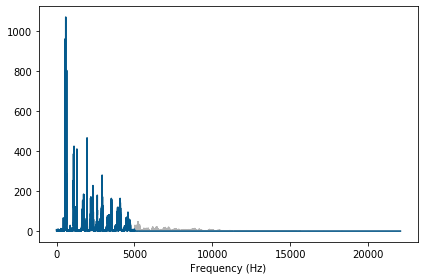## ThinkDSP¶

This notebook contains code examples from Chapter 1: Sounds and Signals

## Think DSP module¶

thinkdsp is a module that accompanies Think DSP and provides classes and functions for working with signals.

In :
# Get thinkdsp.py

import os

if not os.path.exists('thinkdsp.py'):
!wget https://github.com/AllenDowney/ThinkDSP/raw/master/code/thinkdsp.py


## Signals¶

Instantiate cosine and sine signals.

In :
from thinkdsp import CosSignal, SinSignal

cos_sig = CosSignal(freq=440, amp=1.0, offset=0)
sin_sig = SinSignal(freq=880, amp=0.5, offset=0)


Plot the sine and cosine signals. By default, plot plots three periods.

In :
from thinkdsp import decorate

cos_sig.plot()
decorate(xlabel='Time (s)')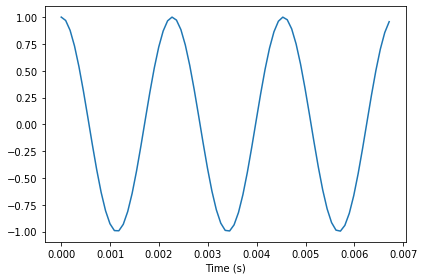Here's the sine signal.

In :
sin_sig.plot()
decorate(xlabel='Time (s)')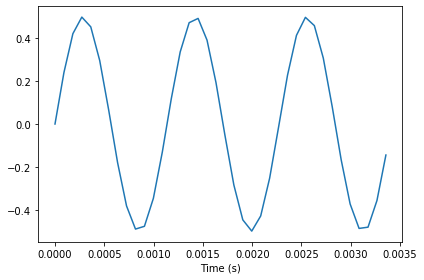Notice that the frequency of the sine signal is doubled, so the period is halved.

The sum of two signals is a SumSignal.

In :
mix = sin_sig + cos_sig
mix

Out:
<thinkdsp.SumSignal at 0x7fc6b8436160>

Here's what it looks like.

In :
mix.plot()
decorate(xlabel='Time (s)')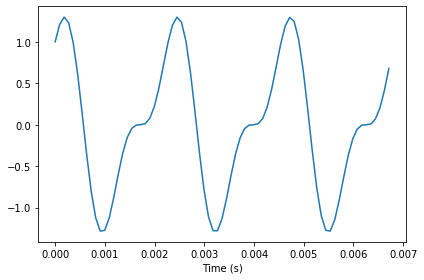## Waves¶

A Signal represents a mathematical function defined for all values of time. If you evaluate a signal at a sequence of equally-spaced times, the result is a Wave. framerate is the number of samples per second.

In :
wave = mix.make_wave(duration=0.5, start=0, framerate=11025)
wave

Out:
<thinkdsp.Wave at 0x7fc6b83912b0>

IPython provides an Audio widget that can play a wave.

In :
from IPython.display import Audio
audio = Audio(data=wave.ys, rate=wave.framerate)
audio

Out:

Wave also provides make_audio(), which does the same thing:

In :
wave.make_audio()

Out:

The ys attribute is a NumPy array that contains the values from the signal. The interval between samples is the inverse of the framerate.

In :
print('Number of samples', len(wave.ys))
print('Timestep in ms', 1 / wave.framerate * 1000)

Number of samples 5512
Timestep in ms 0.09070294784580499


Signal objects that represent periodic signals have a period attribute.

Wave provides segment, which creates a new wave. So we can pull out a 3 period segment of this wave.

In :
period = mix.period
segment = wave.segment(start=0, duration=period*3)
period

Out:
0.0022727272727272726

Wave provides plot

In :
segment.plot()
decorate(xlabel='Time (s)')normalize scales a wave so the range doesn't exceed -1 to 1.

apodize tapers the beginning and end of the wave so it doesn't click when you play it.

In :
wave.normalize()
wave.apodize()
wave.plot()
decorate(xlabel='Time (s)')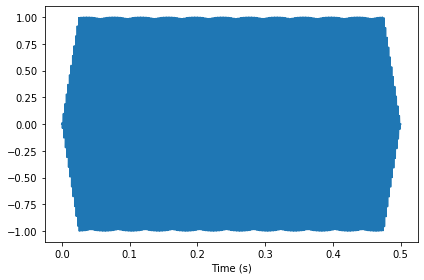You can write a wave to a WAV file.

In :
wave.write('temp.wav')

Writing temp.wav


wave.write writes the wave to a file so it can be used by an exernal player.

In :
from thinkdsp import play_wave

play_wave(filename='temp.wav', player='aplay')


read_wave reads WAV files. The WAV examples in the book are from freesound.org. In the contributors section of the book, I list and thank the people who uploaded the sounds I use.

In :
from thinkdsp import read_wave


In :
wave.make_audio()

Out:

I pulled out a segment of this recording where the pitch is constant. When we plot the segment, we can't see the waveform clearly, but we can see the "envelope", which tracks the change in amplitude during the segment.

In :
start = 1.2
duration = 0.6
segment = wave.segment(start, duration)
segment.plot()
decorate(xlabel='Time (s)')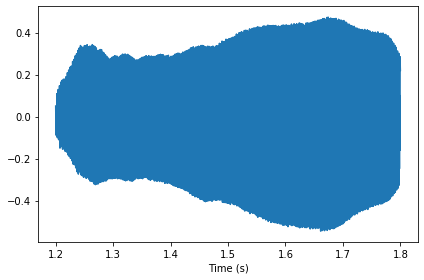## Spectrums¶

Wave provides make_spectrum, which computes the spectrum of the wave.

In :
spectrum = segment.make_spectrum()


Spectrum provides plot

In :
spectrum.plot()
decorate(xlabel='Frequency (Hz)')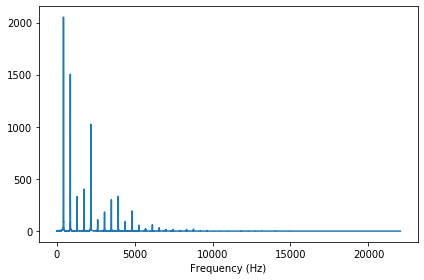The frequency components above 10 kHz are small. We can see the lower frequencies more clearly by providing an upper bound:

In :
spectrum.plot(high=10000)
decorate(xlabel='Frequency (Hz)')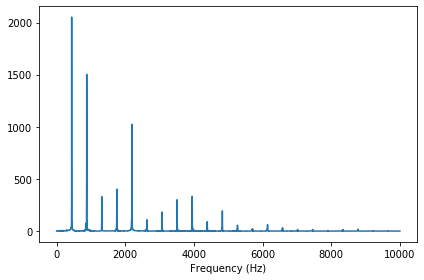Spectrum provides low_pass, which applies a low pass filter; that is, it attenuates all frequency components above a cutoff frequency.

In :
spectrum.low_pass(3000)


The result is a spectrum with fewer components.

In :
spectrum.plot(high=10000)
decorate(xlabel='Frequency (Hz)')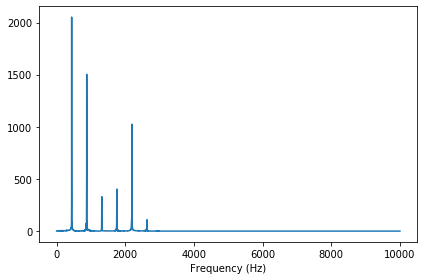We can convert the filtered spectrum back to a wave:

In :
filtered = spectrum.make_wave()


And then normalize it to the range -1 to 1.

In :
filtered.normalize()


Before playing it back, I'll apodize it (to avoid clicks).

In :
filtered.apodize()
filtered.plot()
decorate(xlabel='Time (s)')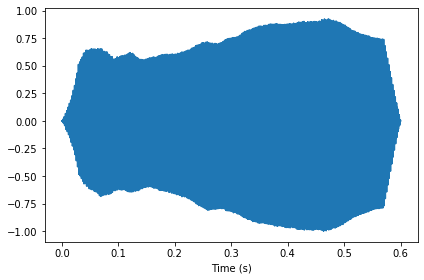And I'll do the same with the original segment.

In :
segment.normalize()
segment.apodize()
segment.plot()
decorate(xlabel='Time (s)')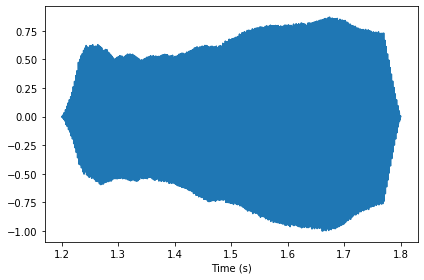Finally, we can listen to the original segment and the filtered version.

In :
segment.make_audio()

Out:
In :
filtered.make_audio()

Out:

The original sounds more complex, with some high-frequency components that sound buzzy. The filtered version sounds more like a pure tone, with a more muffled quality. The cutoff frequency I chose, 3000 Hz, is similar to the quality of a telephone line, so this example simulates the sound of a violin recording played over a telephone.

## Interaction¶

The following example shows how to use interactive IPython widgets.

In :
import matplotlib.pyplot as plt
from IPython.display import display

def filter_wave(wave, start, duration, cutoff):
"""Selects a segment from the wave and filters it.

Plots the spectrum and displays an Audio widget.

wave: Wave object
start: time in s
duration: time in s
cutoff: frequency in Hz
"""
segment = wave.segment(start, duration)
spectrum = segment.make_spectrum()

spectrum.plot(color='0.7')
spectrum.low_pass(cutoff)
spectrum.plot(color='#045a8d')
decorate(xlabel='Frequency (Hz)')
plt.show()

audio = spectrum.make_wave().make_audio()
display(audio)


Adjust the sliders to control the start and duration of the segment and the cutoff frequency applied to the spectrum.

In :
from ipywidgets import interact, fixed